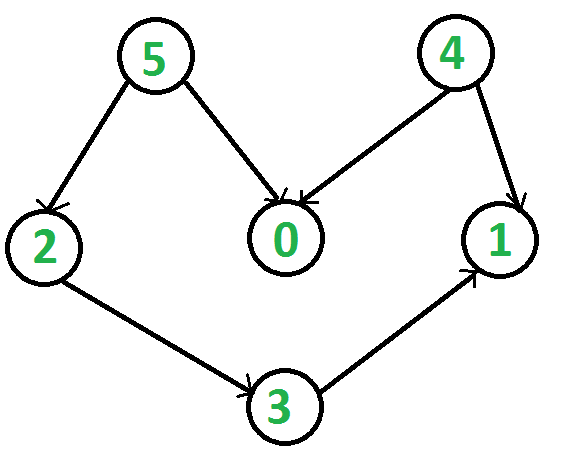Related Articles

# C++ Program for Topological Sorting

• Difficulty Level : Expert
• Last Updated : 06 Dec, 2018

Topological sorting for Directed Acyclic Graph (DAG) is a linear ordering of vertices such that for every directed edge uv, vertex u comes before v in the ordering. Topological Sorting for a graph is not possible if the graph is not a DAG.

For example, a topological sorting of the following graph is “5 4 2 3 1 0”. There can be more than one topological sorting for a graph. For example, another topological sorting of the following graph is “4 5 2 3 1 0”. The first vertex in topological sorting is always a vertex with in-degree as 0 (a vertex with no in-coming edges).`// A C++ program to print topological sorting of a DAG``#include ``#include ``#include ``using` `namespace` `std;`` ` `// Class to represent a graph``class` `Graph {``    ``int` `V; ``// No. of vertices'`` ` `    ``// Pointer to an array containing adjacency listsList``    ``list<``int``>* adj;`` ` `    ``// A function used by topologicalSort``    ``void` `topologicalSortUtil(``int` `v, ``bool` `visited[], stack<``int``>& Stack);`` ` `public``:``    ``Graph(``int` `V); ``// Constructor`` ` `    ``// function to add an edge to graph``    ``void` `addEdge(``int` `v, ``int` `w);`` ` `    ``// prints a Topological Sort of the complete graph``    ``void` `topologicalSort();``};`` ` `Graph::Graph(``int` `V)``{``    ``this``->V = V;``    ``adj = ``new` `list<``int``>[V];``}`` ` `void` `Graph::addEdge(``int` `v, ``int` `w)``{``    ``adj[v].push_back(w); ``// Add w to v’s list.``}`` ` `// A recursive function used by topologicalSort``void` `Graph::topologicalSortUtil(``int` `v, ``bool` `visited[],``                                ``stack<``int``>& Stack)``{``    ``// Mark the current node as visited.``    ``visited[v] = ``true``;`` ` `    ``// Recur for all the vertices adjacent to this vertex``    ``list<``int``>::iterator i;``    ``for` `(i = adj[v].begin(); i != adj[v].end(); ++i)``        ``if` `(!visited[*i])``            ``topologicalSortUtil(*i, visited, Stack);`` ` `    ``// Push current vertex to stack which stores result``    ``Stack.push(v);``}`` ` `// The function to do Topological Sort. It uses recursive``// topologicalSortUtil()``void` `Graph::topologicalSort()``{``    ``stack<``int``> Stack;`` ` `    ``// Mark all the vertices as not visited``    ``bool``* visited = ``new` `bool``[V];``    ``for` `(``int` `i = 0; i < V; i++)``        ``visited[i] = ``false``;`` ` `    ``// Call the recursive helper function to store Topological``    ``// Sort starting from all vertices one by one``    ``for` `(``int` `i = 0; i < V; i++)``        ``if` `(visited[i] == ``false``)``            ``topologicalSortUtil(i, visited, Stack);`` ` `    ``// Print contents of stack``    ``while` `(Stack.empty() == ``false``) {``        ``cout << Stack.top() << ``" "``;``        ``Stack.pop();``    ``}``}`` ` `// Driver program to test above functions``int` `main()``{``    ``// Create a graph given in the above diagram``    ``Graph g(6);``    ``g.addEdge(5, 2);``    ``g.addEdge(5, 0);``    ``g.addEdge(4, 0);``    ``g.addEdge(4, 1);``    ``g.addEdge(2, 3);``    ``g.addEdge(3, 1);`` ` `    ``cout << ``"Following is a Topological Sort of the given graph n"``;``    ``g.topologicalSort();`` ` `    ``return` `0;``}`
Output:
```Following is a Topological Sort of the given graph n5 4 2 3 1 0
```

Please refer complete article on Topological Sorting for more details!

Want to learn from the best curated videos and practice problems, check out the C++ Foundation Course for Basic to Advanced C++ and C++ STL Course for the language and STL. To complete your preparation from learning a language to DS Algo and many more, please refer Complete Interview Preparation Course.

My Personal Notes arrow_drop_up